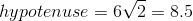# High School Math : Quadrilaterals

## Example Questions

### Example Question #1 : How To Find The Area Of A Rectangle

A rectangle has a perimeter of 40 inches.  It is 3 times as long as it is wide.  What is the area of the rectangle in square inches?

75

45

60

86

75

Explanation:

The width of the rectangle is w, therefore the length is 3w.  The perimeter, P, can then be described as P = w + w + 3w +3w

40 = 8w

w = 5

width = 5, length = 3w = 15

A = 5*15 = 75 square inches

### Example Question #5 : How To Find The Area Of A Rectangle

Angela is carpeting a rectangular conference room that measures 20 feet by 30 feet. If carpet comes in rectangular pieces that measures 5 feet by 4 feet, how many carpet pieces will she need to carpet the entire room?

30

31

600

20

29

30

Explanation:

First, we need to find the area of the room. Because the room is rectangular, we can multiply 20 feet by 30 feet, which is 600 square feet. Next, we need to know how much space one carpet piece covers. Because the carpet pieces are also rectangular, we can multiply 4 feet by 5 feet to get 20 feet. To determine how many pieces of carpet Angela will need, we must divide the total square footage of the room (600 feet) by the square footage covered by one carpet piece (20 feet). 600 divided by 20 is 30, so Angela will need 30 carpet pieces to carpet the entire room.

### Example Question #6 : How To Find The Area Of A Rectangle

If the width of a rectangle is 8 inches, and the length is half the width, what is the area of the rectangle in square inches?

12

32

16

64

20

32

Explanation:

the length of the rectangle is half the width, and the width is 8, so the length must be half of 8, which is 4.

The area of the rectangle can be determined from multiplying length by width, so,

4 x 8 = 32 inches squared

### Example Question #7 : How To Find The Area Of A Rectangle

If Mrs. Stietz has a patio that measures 96 inches by 72 inches and she wants to cover it with stone tiles that measure one foot by half a foot, what is the minimum number of tiles she needs to cover the patio?

6912

48

96

12

14

96

Explanation:

96. Converting the dimensions of the tiles to inches, they each measure 12 inches by 6 inches.  This means that there need to be 8 tiles to span the length of the patio, and 6 tiles to span the width of the patio.  She needs to cover the entire area, so we can multiply 8 times 12 to get 96, the number of tiles she needs for the patio.

### Example Question #6 : How To Find The Area Of A Rectangle

The front façade of a building is 100 feet tall and 40 feet wide.  There are eight floors in the building, and each floor has four glass windows that are 8 feet wide and 6 feet tall along the front façade.  What is the total area of the glass in the façade?

2464 ft2

768 ft2

1536 ft2

192 ft2

1536 ft2

1536 ft2

Explanation:

Glass Area per Window = 8 ft x 6 ft = 48 ft2

Total Number of Windows = Windows per Floor * Number of Floors = 4 * 8 = 32 windows

Total Area of Glass = Area per Window * Total Number of Windows = 48 * 32 = 1536 ft2

### Example Question #1 : How To Find The Area Of A Rectangle

Mark is making a plan to build a rectangular garden.  He has 160 feet of fence to form the outside border of the garden.  He wants the dimensions to look like the plan outlined below: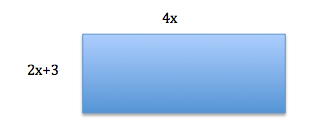What is the area of the garden, rounded to the nearest square foot?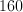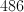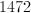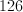Explanation:

Perimeter:  Sum of the sides:

4x + 4x + 2x+8 +2x+8 = 160

12x + 6 = 160

12x = 154

x =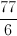Therefore, the short side of the rectangle is going to be: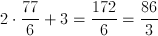And the long side is going to be: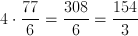The area of the rectangle is going to be as follows:

Area = lw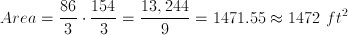### Example Question #191 : Geometry

Two circles of a radius of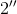each sit inside a square with a side length of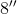.  If the circles do not overlap, what is the area outside of the circles, but within the square?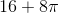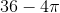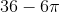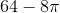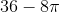Explanation:

The area of a square =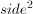The area of a circle is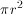Area  = Area of Square2(Area of Circle) =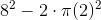### Example Question #198 : Geometry

If the area Rectangle A islarger than Rectangle B and the sides of Rectangle A areand, what is the area of Rectangle B?Explanation: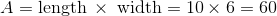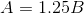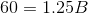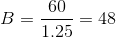### Example Question #231 : Plane Geometry

Erin is getting ready to plant her tulip garden. She wants to plant two tulips per square foot of garden. If her rectangular garden is enclosed by 24 feet of fencing, and the length of the fence is twice as long as its width, how many tulips will Erin plant?

48

32

24

64

16

64

Explanation:

We know that the following represents the formula for the perimeter of a rectangle:In this particular case, we are told that the length of the fence is twice as long as the width. We can write this as the following expression:Use this information to substitute in a variable for the length that matches the variable for width in our perimeter equation.We also know that the length is two times the width; therefore, we can write the following:The area of a rectangle is found by using this formula:The area of the garden is 32 square feet. Erin will plant two tulips per square foot; thus, she will plant 64 tulips.

### Example Question #1 : How To Find The Length Of The Diagonal Of A Square

What is the length of a diagonal of a square with a side length? Round to the nearest tenth.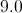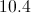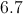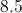Explanation:

A square is comprised of two 45-45-90 right triangles. The hypotenuse of a 45-45-90 right triangle follows the rule below, whereis the length of the sides.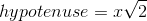In this instance,is equal to 6.